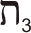Historical Archive of the Bible Wheel Site

The Bible Wheel had been debunked by its author.
Read all about it: Debunking Myself: What A Long Strange Trip It's Been

Recent Blog ArticlesGreat is our Lord, and of great power: his understanding is infinite.

Psalm 147:4

The circular structure of the Wheel is most naturally described using modular arithmetic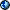, the mathematics of cyclical number patterns. For example, the books found on the first Spoke are the 1st, 23rd, and 45th books of the Canon. These numbers all leave the same remainder, namely 1, when divided by 22. This is because they are all 1 plus a multiple of 22. Likewise, the ordinal numbers of the books on the second Spoke always leave a remainder of 2 when divided by 22. This is because they are all 2 plus a multiple of 22 + 2. These patterns are displayed in the table below.

Mathematicians describe such numbers by saying they are equal or congrugent Modulo 22. These ideas help see the structure of the Bible Wheel because the ordinal number of each book relates to its Spoke and Cycle numbers through this simple formula:

Book Number = Spoke + (Cycle - 1) x 22

This table gives a few examples of how this works:

 Spoke 1 Spoke 2 ... Spoke 22 Cycle 1 1 = 1 + 0 x 22 2 = 2 + 0 x 22 ... 22 = 22 + 0 x 22 Cycle 2 23 =  1 + 1 x 22 24 =  2 + 1 x 22 ... 44 = 22 + 1 x 22 Cycle 3 45 =  1 + 2 x 22 46 =  2 + 2 x 22 ... 66 = 22 + 2 x 22

A very useful and compact notation that brings forth many patterns from God's Word is found when we denote a book's ordinal number using a Spoke Number (or Letter) with a subscript indicating the Cycle Number:

Book Number = SpokeCycle

This table gives a few examples of this notation:

 Spoke 1 Spoke 2 ... Spoke 22 Cycle 1 1 =  11 2 =  21 ... 22 = 221 Cycle 2 23 =  12 24 =  22 ... 44 = 222 Cycle 3 45 =  13 46 =  23 ... 66 = 223

This pattern can be generalized so that any number can be written this way by allowing the Cycle number to increase indefinitely. For example, this notation displays the natural relation between the Number 22 and all repeating three-digit numbers:

 111 = 1 +   5 x 22 = 16 222 = 2 + 10 x 22 = 211 333 = 3 + 15 x 22 = 316

In general, for 0 < n < 10, we have: nnn = n5n+1

This notation can be extended to use the letters to represent the Spokes. I like this notation for personal use because it makes the links between the geometry and the alphabet very clear, but I don't use it much on the site because most people are not familiar with the Hebrew alphabet, and I don't want to place any stumblingblocks in their way. But here is a table that shows how modular notation works with the alphabet:

 Spoke 1 Spoke 2 ... Spoke 22 Cycle 1 1 = 11 =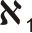2 = 21 =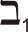... 22 = 221 =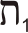Cycle 2 23 = 12 =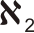24 = 22 =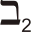... 44 = 222 =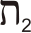Cycle 3 45 = 13 =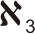46 = 23 =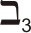... 66 = 223 =